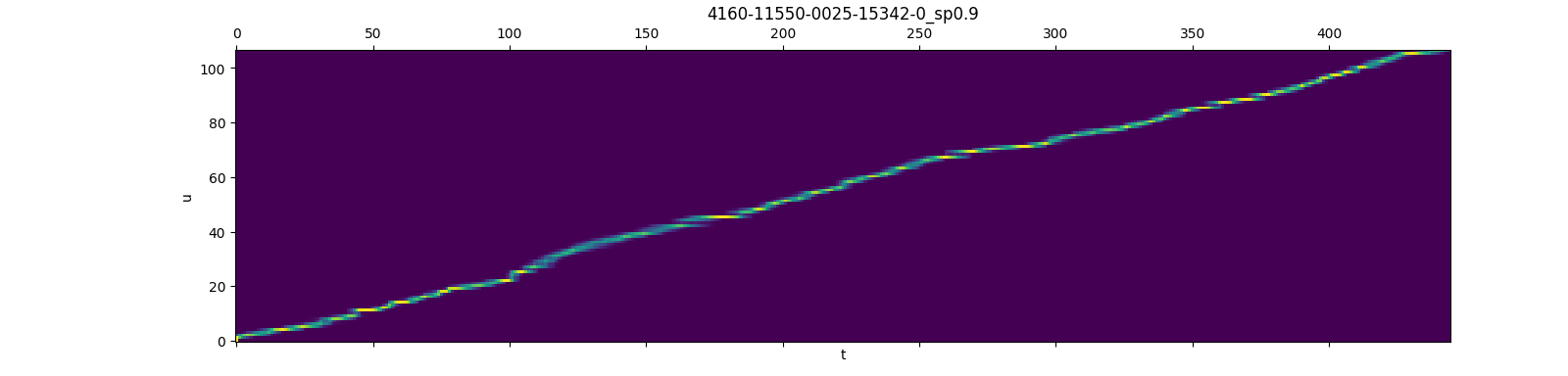This project implements a method for faster and more memory-efficient RNN-T loss computation, called `pruned rnnt`.

Note: There is also a fast RNN-T loss implementation in k2 project, which shares the same code here. We make `fast_rnnt` a stand-alone project in case someone wants only this rnnt loss.

## How does the pruned-rnnt work ?

We first obtain pruning bounds for the RNN-T recursion using a simple joiner network that is just an addition of the encoder and decoder, then we use those pruning bounds to evaluate the full, non-linear joiner network.

The picture below display the gradients (obtained by `rnnt_loss_simple` with `return_grad=true`) of lattice nodes, at each time frame, only a small set of nodes have a non-zero gradient, which justifies the pruned RNN-T loss, i.e., putting a limit on the number of symbols per frame.This picture is taken from here

## Installation

You can install it via `pip`:

``````pip install fast_rnnt
``````

You can also install from source:

``````git clone https://github.com/danpovey/fast_rnnt.git
cd fast_rnnt
python setup.py install
``````

To check that `fast_rnnt` was installed successfully, please run

``````python3 -c "import fast_rnnt; print(fast_rnnt.__version__)"
``````

which should print the version of the installed `fast_rnnt`, e.g., `1.0`.

### How to display installation log ?

Use

``````pip install --verbose fast_rnnt
``````

### How to reduce installation time ?

Use

``````export FT_MAKE_ARGS="-j"
pip install --verbose fast_rnnt
``````

It will pass `-j` to `make`.

### Which version of PyTorch is supported ?

It has been tested on PyTorch >= 1.5.0.

Note: The cuda version of the Pytorch should be the same as the cuda version in your environment, or it will cause a compilation error.

### How to install a CPU version of `fast_rnnt` ?

Use

``````export FT_CMAKE_ARGS="-DCMAKE_BUILD_TYPE=Release -DFT_WITH_CUDA=OFF"
export FT_MAKE_ARGS="-j"
pip install --verbose fast_rnnt
``````

It will pass `-DCMAKE_BUILD_TYPE=Release -DFT_WITH_CUDA=OFF` to `cmake`.

## Usage

### For rnnt_loss_simple

This is a simple case of the RNN-T loss, where the joiner network is just addition.

Note: termination_symbol plays the role of blank in other RNN-T loss implementations, we call it termination_symbol as it terminates symbols of current frame.

```am = torch.randn((B, T, C), dtype=torch.float32)
lm = torch.randn((B, S + 1, C), dtype=torch.float32)
symbols = torch.randint(0, C, (B, S))
termination_symbol = 0

boundary = torch.zeros((B, 4), dtype=torch.int64)
boundary[:, 2] = target_lengths
boundary[:, 3] = num_frames

loss = fast_rnnt.rnnt_loss_simple(
lm=lm,
am=am,
symbols=symbols,
termination_symbol=termination_symbol,
boundary=boundary,
reduction="sum",
)```

### For rnnt_loss_smoothed

The same as `rnnt_loss_simple`, except that it supports `am_only` & `lm_only` smoothing that allows you to make the loss-function one of the form:

``````      lm_only_scale * lm_probs +
am_only_scale * am_probs +
(1-lm_only_scale-am_only_scale) * combined_probs
``````

where `lm_probs` and `am_probs` are the probabilities given the lm and acoustic model independently.

```am = torch.randn((B, T, C), dtype=torch.float32)
lm = torch.randn((B, S + 1, C), dtype=torch.float32)
symbols = torch.randint(0, C, (B, S))
termination_symbol = 0

boundary = torch.zeros((B, 4), dtype=torch.int64)
boundary[:, 2] = target_lengths
boundary[:, 3] = num_frames

loss = fast_rnnt.rnnt_loss_smoothed(
lm=lm,
am=am,
symbols=symbols,
termination_symbol=termination_symbol,
lm_only_scale=0.25,
am_only_scale=0.0
boundary=boundary,
reduction="sum",
)```

### For rnnt_loss_pruned

`rnnt_loss_pruned` can not be used alone, it needs the gradients returned by `rnnt_loss_simple/rnnt_loss_smoothed` to get pruning bounds.

```am = torch.randn((B, T, C), dtype=torch.float32)
lm = torch.randn((B, S + 1, C), dtype=torch.float32)
symbols = torch.randint(0, C, (B, S))
termination_symbol = 0

boundary = torch.zeros((B, 4), dtype=torch.int64)
boundary[:, 2] = target_lengths
boundary[:, 3] = num_frames

# rnnt_loss_simple can be also rnnt_loss_smoothed
lm=lm,
am=am,
symbols=symbols,
termination_symbol=termination_symbol,
boundary=boundary,
reduction="sum",
)
s_range = 5  # can be other values
ranges = fast_rnnt.get_rnnt_prune_ranges(
boundary=boundary,
s_range=s_range,
)

am_pruned, lm_pruned = fast_rnnt.do_rnnt_pruning(am=am, lm=lm, ranges=ranges)

logits = model.joiner(am_pruned, lm_pruned)
pruned_loss = fast_rnnt.rnnt_loss_pruned(
logits=logits,
symbols=symbols,
ranges=ranges,
termination_symbol=termination_symbol,
boundary=boundary,
reduction="sum",
)```

You can also find recipes here that uses `rnnt_loss_pruned` to train a model.

### For rnnt_loss

The `unprund rnnt_loss` is the same as `torchaudio rnnt_loss`, it produces same output as torchaudio for the same input.

```logits = torch.randn((B, S, T, C), dtype=torch.float32)
symbols = torch.randint(0, C, (B, S))
termination_symbol = 0

boundary = torch.zeros((B, 4), dtype=torch.int64)
boundary[:, 2] = target_lengths
boundary[:, 3] = num_frames

loss = fast_rnnt.rnnt_loss(
logits=logits,
symbols=symbols,
termination_symbol=termination_symbol,
boundary=boundary,
reduction="sum",
)```

## Benchmarking

The repo compares the speed and memory usage of several transducer losses, the summary in the following table is taken from there, you can check the repository for more details.

Note: As we declared above, `fast_rnnt` is also implemented in k2 project, so `k2` and `fast_rnnt` are equivalent in the benchmarking.

Name Average step time (us) Peak memory usage (MB)
torchaudio 601447 12959.2
fast_rnnt(unpruned) 274407 15106.5
fast_rnnt(pruned) 38112 2647.8
optimized_transducer 567684 10903.1
warprnnt_numba 229340 13061.8
warp-transducer 210772 13061.8

View Github